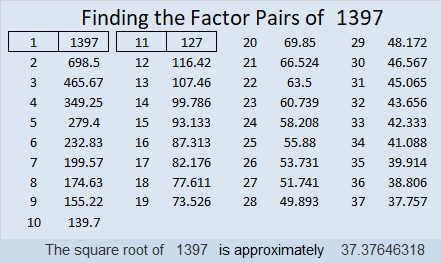# 1397 and Level 4

I bet you know enough multiplication facts to get this puzzle started. Once you’ve started it, you might as well finish it. You will feel so clever when you do!Print the puzzles or type the solution in this excel file: 12 Factors 1389-1403

Now I’ll write a little bit about the puzzle number, 1397:

• 1397 is a composite number.
• Prime factorization: 1397 = 11 × 127
• 1397 has no exponents greater than 1 in its prime factorization, so √1397 cannot be simplified.
• The exponents in the prime factorization are 1, and 1. Adding one to each exponent and multiplying we get (1 + 1)(1 + 1) = 2 × 2 = 4. Therefore 1397 has exactly 4 factors.
• The factors of 1397 are outlined with their factor pair partners in the graphic below.1397 is the difference of two squares two different ways:
699² – 698² = 1397
69² – 58² = 1397

This site uses Akismet to reduce spam. Learn how your comment data is processed.